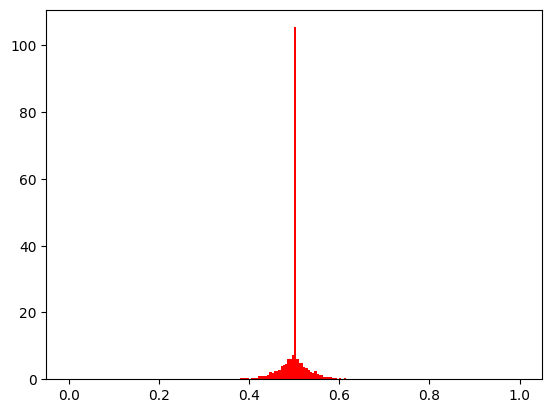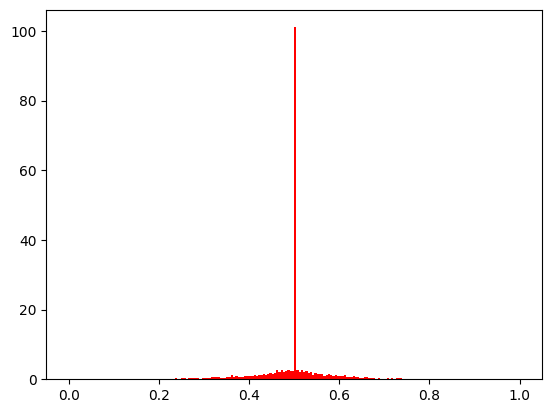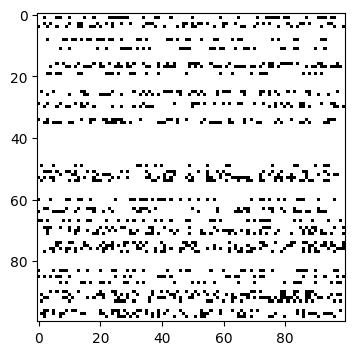Version: 0.5.0

# Mutation¶

## Polynomial Mutation (‘real_pm’, ‘int_pm’)¶

Details about the mutation can be found in . This mutation follows the same probability distribution as the simulated binary crossover.

:

from pymoo.interface import mutation
from pymoo.factory import get_mutation
import numpy as np
import matplotlib.pyplot as plt

def show(eta_mut):
a = np.full((5000, 1), 0.5)
off = mutation(get_mutation("real_pm", eta=eta_mut, prob=1.0), a)

plt.hist(off, range=(0,1), bins=200, density=True, color="red")
plt.show()

show(30):

show(10)Basically, the same can be applied to discrete variables as well:

:


def show(eta_mut):
a = np.full((10000, 1), 0)
off = mutation(get_mutation("int_pm", eta=eta_mut, prob=1.0), a, xl=-20, xu=20)

plt.hist(off, range=(-20, 20), bins=40, density=True, color="red")
plt.show()

show(30)## Bitflip Mutation (‘bin_bitflip’)¶

The bitlip mutation randomly flips a bit.

:

def show(M):
plt.figure(figsize=(4,4))
plt.imshow(M, cmap='Greys',  interpolation='nearest')
plt.show()

a = np.full((100,100), False)
mut = mutation(get_mutation("bin_bitflip", prob=0.1), a)

show(a != mut)## API¶

pymoo.factory.get_mutation(name, kwargs)

A convenience method to get a mutation object just by providing a string.

Parameters
name{ ‘none’, ‘real_pm’, ‘int_pm’, ‘bin_bitflip’, ‘perm_inv’ }

Name of the mutation.

kwargsdict

Dictionary that should be used to call the method mapped to the mutation factory function.

Returns
classMutation

An mutation object based on the string. None if the mutation was not found.

pymoo.core.mutation.Mutation()None